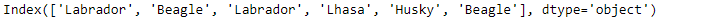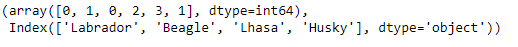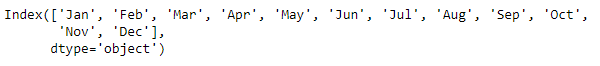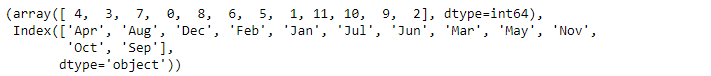Related Articles

# Python | Pandas Index.factorize()

• Last Updated : 17 Dec, 2018

Python is a great language for doing data analysis, primarily because of the fantastic ecosystem of data-centric python packages. Pandas is one of those packages and makes importing and analyzing data much easier.

Pandas` Index.factorize()` function encode the object as an enumerated type or categorical variable. This method is useful for obtaining a numeric representation of an array when all that matters is identifying distinct values. factorize is available as both a top-level function pandas.factorize(), and as a method Series.factorize() and Index.factorize().

Syntax: Index.factorize(sort=False, na_sentinel=-1)

Parameters :
sort : Sort uniques and shuffle labels to maintain the relationship.

Returns : An integer ndarray that’s an indexer into uniques. uniques.take(labels) will have the same values as values.

Example #1: Use `Index.factorize()` function to encode the given Index values into categorical form.

 `# importing pandas as pd``import` `pandas as pd`` ` `# Creating the Index``idx ``=` `pd.Index([``'Labrador'``, ``'Beagle'``, ``'Labrador'``,``                     ``'Lhasa'``, ``'Husky'``, ``'Beagle'``])`` ` `# Print the Index``idx`

Output :Let’s factorize the given Index.

 `# convert it into categorical values.``idx.factorize()`

Output :As we can see in the output, the `Index.factorize()` function has converted each label in the Index to a category and has assigned them numerical values.

Example #2: Use `Index.factorize()` function to factorize the index values based on their sorted order sequence.

 `# importing pandas as pd``import` `pandas as pd`` ` `# Creating the Index``idx ``=` `pd.Index([``'Jan'``, ``'Feb'``, ``'Mar'``, ``'Apr'``, ``'May'``, ``'Jun'``,``               ``'Jul'``, ``'Aug'``, ``'Sep'``, ``'Oct'``, ``'Nov'``, ``'Dec'``])`` ` `# Print the Index``idx`

Output :Let’s factorize it based on sorted order. Numerical values are assigned only after the sorting of the values in the Index.

 `# Factorize the sorted labels``idx.factorize(sort ``=` `True``)`

Output :As we can see in the output, sorting has been performed on the Index values before assigning them numerical values.

Attention geek! Strengthen your foundations with the Python Programming Foundation Course and learn the basics.

To begin with, your interview preparations Enhance your Data Structures concepts with the Python DS Course. And to begin with your Machine Learning Journey, join the Machine Learning – Basic Level Course

My Personal Notes arrow_drop_up